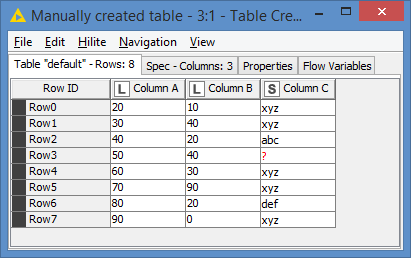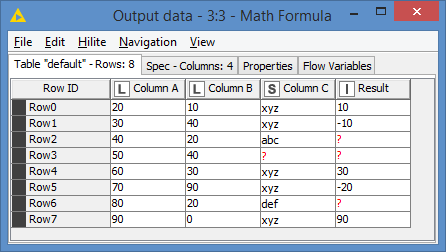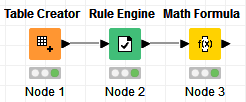# Condition based Math issue

Hi,

I have a dataset where I would like to perform a subtraction of two column values (number long) based on condition of 3rd column (String). Output is a number.
Tried using the rule engine node, but got errorsLets say, Column A and Column B are numbers and Column C is string.
Column C = “xyz” => Column A - Column B

Would anyone provide suggestions/insights on how to do this?

Thank you.

The Rule Engine does not support these kind of operations. You are better off with other nodes like the Column Expression.

For example, in its basic form:

``````if (column("column3").equals("xyz")) {
column("column1") - column("column2")
}  else {
null
}
``````

If you want a more convenant approach when dealing with multiple rules for example, you could still use a Rule Engine as a first step to create a true/false boolean. Next, you apply the calculation for only those rows that are `true`.

To illustrate,

``````\$column3\$ = "xyz" => TRUE
TRUE => FALSE
``````

With associated updated evaluation logic.

``````if (column("prediction") ==  true) {
column("column1") - column("column2")
}  else {
null
}
``````

Hope this helps!

5 Likes

Hi @rohinipe ,

As @ArjenEX mentioned, the Rule Engine does not support these kind of operations. The Rule Engine allows you to implement the conditions, but the values can only be static values, so you can’t do subtraction of 2 columns.

Column Expressions is an option. It’s very flexible in that you can code whatever you want to do there.

However, the Column Expressions node is a slow node. Obviously if you are going to run it on a small dataset, it might not matter, but keep in mind that it’s a slow node, and because of this, I would rather implement this via the Math Formula node instead. (I use Column Expressions only if there is no other better way)

You will still need to use the Rule Engine, and we can simply set the results of the rule to some numeric values that we can use in the Math Formula as identifier.

For example, we can do this:

``````\$Column C\$ = "xyz" => 1
TRUE => 0
``````

And let’s say for the demo, I have this data as input:After applying the Rule Engine, I have now identified which rows need the operation (Column A - Column B) based on Column C having “xyz” as value:The good thing about the Math Formula node is that it does of course allow you to do operations, but it also support if conditions in the form of: `if (cond, trueval, falseval)`

Since you have only shown us what the results should be when Column C = “xyz” but not what the results should be when Column C is not “xyz”, I will assume that the results should be Null (empty) in this case.

So, with the above assumption, one way is to use the if like this:

`if(\$Result\$ == 1, \$Column A\$ - \$Column B\$, \$Result\$/0)`

NOTE: I’m doing `\$Result\$/0` as a trick to generate a null (empty) results.

And that’s the results that I get:Now, the reason why I assigned specifically 1 and 0 in the Rule Engine is that there’s an even smarter way (and faster way) to do this operation.

You can simply just run this operation, without the if(), which makes it run even faster:

`(\$Column A\$ - \$Column B\$) / \$Result\$`

And I get the same results as with the if():The workflow is simply like this:4 Likes

Thank you so much @ArjenEX @bruno29a . This solution helped me solve the issue

This topic was automatically closed 90 days after the last reply. New replies are no longer allowed.